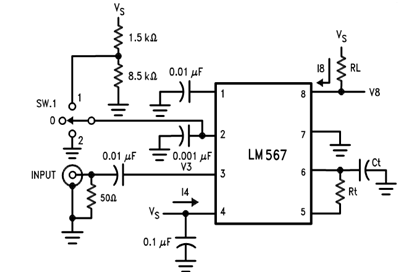# Voltage Controlled Oscillator – Usage of VCO, Working and Application

### What is Voltage Controlled Oscillator?

A voltage-controlled oscillator is an oscillator with an output signal whose output can be varied over a range, which is controlled by the input DC voltage. It is an oscillator whose output frequency is directly related to the voltage at its input. The oscillation frequency varies from few hertz to hundreds of GHz. By varying the input DC voltage, the output frequency of the signal produced is adjusted.

### 2 Types of Voltage Controlled Oscillators

• Harmonic Oscillators: The output is a signal with a sinusoidal waveform. Examples are crystal oscillators and tank oscillators
• Relaxation Oscillators: The output is a signal with a sawtooth or triangular waveform and provides a wide range of operational frequencies. The output frequency depends on the time of charging and discharging of the capacitor.

### Basic Working principle of Sawtooth waveform generator VCO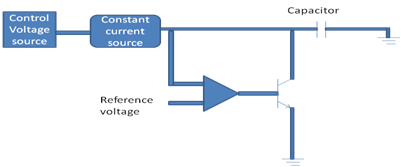For a Voltage controlled oscillator generating a sawtooth waveform, the main component is the capacitor who’s charging and discharging decides the formation of the output waveform. The input is given in form a voltage that can be controlled. This voltage is converted to a current signal and is applied to the capacitor. As the current passes through the capacitor, it starts charging and a voltage starts building across it. As the capacitor charges and the voltage across it increase gradually, the voltage is compared with a reference voltage using a comparator.

When the capacitor voltage exceeds the reference voltage, the comparator generates a high logic output that triggers the transistor, and the capacitor is connected to the ground and starts discharging. Thus the output waveform generated is the representation of the charging and discharging of the capacitor and the frequency is controlled by the input dc voltage.

### Applications of VCO

• Electronic jamming equipment.
• Function generator.
• Production of electronic music, for the production of different types of noise.
• Phase-locked loop.
• Frequency synthesizers, used in communication circuits.

### A Practical VCO – LM566

A practical example of a voltage-controlled oscillator (VCO) is the LM566. The LM566 is a general-purpose VCO that may be used to generate square wave and triangular waveforms as a function input voltage.

The LM566 is specified for operation over 0˚C to 70˚C temperature range. The frequency of which is a linear function of a controlling voltage. The frequency is also controlled by an external resistor and capacitor, whose values control the free-running frequency.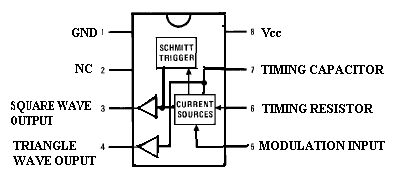#### Pin Description:

• Pin 1: Ground (GND)
• Pin 2: No connection (NC)
• Pin 3: Square wave output
• Pin 4: Triangular wave output
• Pin 5: Modulation input
• Pin 6: Timing resistor
• Pin 7: Timing capacitor
• Pin 8: Vcc

#### Features:

• The maximum operating voltage is 10V to 24V
• High-temperature stability
• Operating temperature is 0˚C to 70˚C
• The frequency can be controlled using current, voltage, resistor or capacitor
• Power dissipation is 300mV
• Excellent power supply rejection

#### Applications:

• Function generator
• Tone generator
• FM modulation
• Frequency shift keying
• Clock generator

### Working of LM566:

The figure shows that the LM566 IC contains current sources to charge and discharge an external capacitor at a rate set by an external resistor R1 and the modulating dc input voltage V.

A 0.001µF capacitor is connected to pin 5 and pin 6. A Schmitt trigger circuit is used to switch the current sources between charging and discharging the capacitor and the triangular voltage produced across the capacitor and square wave from the Schmitt trigger are provided as outputs through buffer amplifiers. Both the output waveforms are buffered so that the output impedance of each is 50 f2. The typical magnitude of the triangular wave and the square wave is 2.4Vpeak to peak and 5.4Vpeak to the peak. The free-running or center-operating frequency, f0 is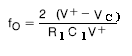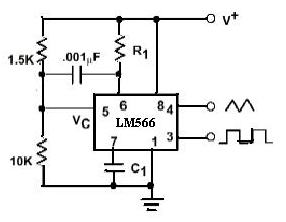### Application of VCO – Phase-Locked Loop

What is the Phase-Locked Loop?

It is an electronic circuit that is used to lock the output frequency of the voltage-controlled oscillator with the desired input frequency by constantly comparing the phase of the input frequency with that of the output frequency of the VCO. The PLL is used to generate a signal, modulate, or demodulate it. They are mainly used in Frequency modulation and Amplitude modulation.  The output frequency of the voltage controlled oscillator is constantly adjusted until it matches with the input frequency.

### How does a Phase Locked Loop works?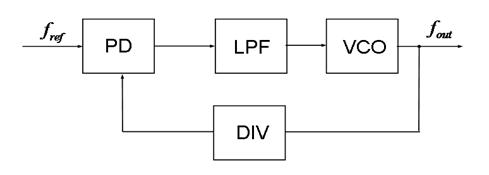In the above block diagram, the PD or Phase detector compares the output frequency with the input reference frequency. In case of any mismatch, the phase detector generates an error signal which is filtered using a low pass filter to remove the noise and this signal is applied to the Voltage-controlled Oscillator to accordingly generate the output frequency. This output frequency is given to the phase detector through a divide by N counter which divides the output frequency by a certain number N.

### A Practical application of PLL – Tone Decoder using LM567

The LM567 is a tone decoder. It is intended to give a saturated transistor switch to ground when the input signal is available. It consists of a voltage-controlled oscillator (VCO) and the phase detector. A voltage-controlled oscillator is to verify the center frequency of the decoder. External components are used to set the center frequency, bandwidth, and output delay.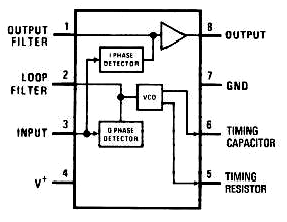The phase detector and VCO form a phase-locked loop (PLL) when the PLL is locked and the input signal amplitude exceeds an internally pre-set threshold, a switch to the ground is activated on the output.

#### Features:

• 20 to 1 frequency range with an external resistor
• Logic compatible output with 100mA current-sinking capability